# Modeling Interest During Construction (IDC) – Excel Project Finance

Share

This is a guest post written by Paramdeep from Pristine. Chandoo.org runs Financial Modeling School program in partnership with Pristine Careers. Visit Financial Modeling School to learn more and sign-up for our newsletter.

Who is not interested in buying a new house? Owning a (at least the first one) house is like a dream come true for most! If you have ever bought (or thought of buying) a house in a building that is yet to be constructed, you would realize that there are clearly two parts of the business for the developer – the construction period (which is when the building is being built for the first 2-3 years) and the operations/ sales period (after the construction, they would sell or lease the building).

As we discussed last time, one of the key aspects of any Infrastructure/ Real Estate Project is the long gestation period of the project. Typically in the construction period the project would utilize all the cash and when the operations/ sales period starts, the costs are almost zero as compared to the revenue being generated from the project.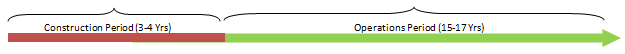### So what’s the big deal about the cash flow structure?

One of biggest concern in the construction period (Lets say it runs for 3 years) is that it consumes all your cash. If my total cost of building the project is going to be USD 30 Million (Spread equally over the three construction years), my Profit and Loss Statement would look something like:

### P&L (all figures in USD Mi)But my building is not yet built (hence I cannot sell it), so I can take this as a Work In Progress (WIP) to my balance sheet (more about this can be learnt from accounting books and we would also be delving into this concept in detail in our training). But one thing is for sure, I would have a cash deficit of USD 10 Mn in the first year and a similar situation would continue for the first three years (till the point construction is complete and you start selling/ operations).

Typically, these projects also have a large amount of debt. For example, if I am expecting to construct the building for USD 30 Mn, I would try to take a bank loan of at least USD 20 Mn.

If you were a bank, the decision making of whether to lend money is taken on the interest and principal repaying capacity. If as a bank I analyze your P&L, I find that you have no cash to pay me my interest and principal! Traditional bankers lend you money ONLY if you can EASILY pay me back my money (typically look at a ratio of (interest + repayment) amount to the cash generated – called coverage ratio). If you can’t do that – I will not lend!

### So how do Banks view this?

As a banker, I understand that you are going to construct for 3 years and that is when you need my money (And would not be able to pay me interest). So I agree to not take interest and principal repayment as cash each year. But I cannot let go of this money!

Think of it as – I let you take additional loan to fund this payment! For example, let us assume:

Interest Rate prevailing: 10% per year

Loan amount in first year: USD 10 Mn

1. So Interest on this loan: USD 1 Mn
2. Now you can’t pay me back, so take additional loan (In first year itself) of USD 1 Mn
3. That means total loan: USD 11 Mn (10 that you originally took and 1 that you took to pay the interest)
4. That means interest is actually USD 1.1 Mn (Instead of the original 1 Mn)
5. That means that effective loan: USSD 11.1 Mn (11 that we had calculated earlier and 0.1 to fund this gap)
6. So interest: USD 1.11 Mn
7. So effective Loan amount: USD 11.11

… and so on

There is a clear circular logic in this concept – My loan changes interest and interest changes loan

### Interpreting the circular logic

Summarizing our thoughts:

• When an asset is developed, and there is a considerable period between the start of a project and its completion, the interest costs related to the construction are generally included in the cost of the asset, that is, the interest cost is capitalized
• The capitalization period ends when the asset is ready for use
• While modeling in excel, Interest During Construction (IDC) introduces a circular loop into the sheet due to the circular references explained below (1-2-3-4)

o Equity and Grant commitments can be either a specific amount, or a certain percentage of the total project funds required (that is, a fixed percentage in the capital structure)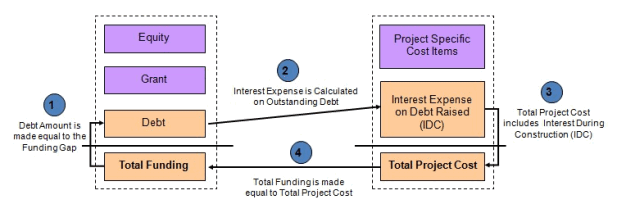### The Case – Modeling Interest During Construction in a typical Real Estate Project

Let us consider the construction period of a project at place X, where government wants to build a hospital.

The costs of the project are stated below: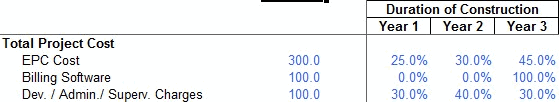The government is ready to provide a grant of USD 50 Mn in the project and the project builder has to infuse equity of USD 100 Mn in the project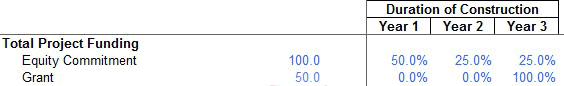The shortfall in the funds can be funded through debt.

A complete model for financing has to be prepared for the construction period.

### The Concept

The basic concept behind the model is pretty simple

### Total cash outflow in a year = Total Cash Inflow in the year

1. So the first step is to calculate the cash outflow in all the years. This cash outflow also includes the cost of paying the interest (which we would not know in the first pass).
2. As a next step, we find the amount available to us through the equity and grants.
3. We know that cash inflow has to be equal to cash outflow for all years.
4. Whatever is the shortfall, we raise debt to fund it.
5. Calculate the cumulative debt
6. We calculate the interest on this debt.
7. Whatever is the interest on the debt, we plug it back in the project cost (and hence introduce the circular logic in the model)

### Step I: Getting the Cash Outflow (Project Costs)

Based on the case, calculate the cash required in each year.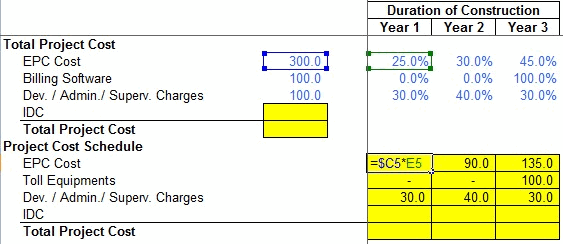We know the costs of each of the items and what should be the contribution in each year. Multiply the values to get the amounts in each year!

In the same step, we add all the costs (Including the Interest During Construction, though we don’t know it right now)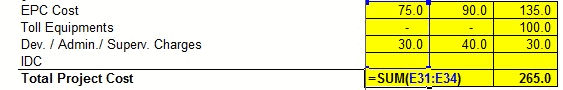### Step II: Getting the Cash Available (through Equity and Grants)

Based on the equity and grant infusion schedule, we calculate the cash inflows### Step III: Cash Inflow = Cash Outflow

Since the cash outflow has to be matched with cash inflow, we make the total project cost in all years equal to funding in the year### Step IV: Fund the shortfall through debt

Since the only source to fund the shortfall is debt, lets raise the debt as the total fund needs less whatever is available through equity and grants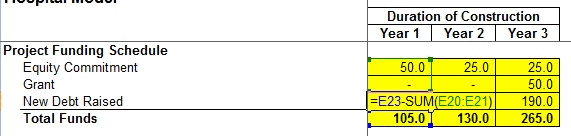### Step V: Calculate the total debt outstanding

Since there is no way that we can pay the debt in the construction time, we make the outstanding debt as the cumulative debt raised (See me use a trick to accumulate!)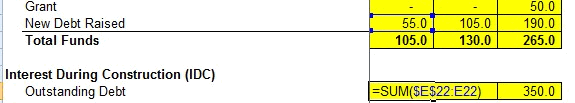### Step VI: Calculate the interest on the debt

Since we have taken money from the bank, we need to pay an interest on it. The interest rate is given to us, let us link the amount to the interest to calculate the interest.### Step VII: The Circular Logic (Plugging back the interest in the project cost)

Since the interest is also a cost of the project (and we are not paying it back to the bank each year), we take it to the project cost.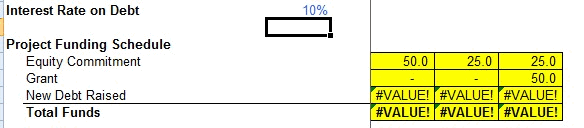Out here, if you notice, excel starts a circular calculation and updates all the values! This can be verified by looking at the bottom left of excel and noticing this sign of “calculate”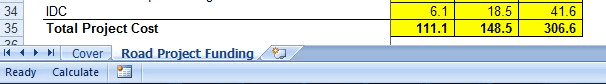### Beware! Circular References can be dangerous!

What we have achieved in this tutorial is one of the most intricate concepts in project finance -Interest During Construction (IDC). We have also used a fairly advanced function in excel – Circular loops. But please note that circular loops in excel is a dangerous tool. If by chance your excel sheet gets an erroneous value, the error would propagate through the model and there is no way for the model to recover back from the error, unless you know where the circular loop is and you delete and go back from there. For example, if I change 10% interest to “ten”,I figure that my model is corrupt (It was expecting a numeric input and I gave a string!). But I can go back to 10%, my model does not go back!!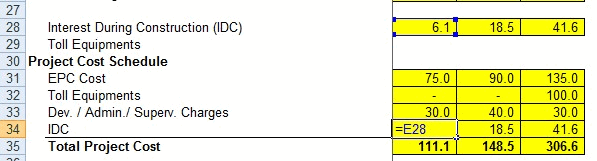I leave it as a homework for you to figure out, how to go back to a stable state!! 🙂

I will give you a trivial solution (close the sheet and open it again) :). You figure out, where the circular loop is and delete those lines and break it to come back!!

In the meanwhile, happy modeling!!

I have created a template for you, where the assumption numbers are given and you have to link the complete model!

You can download the same from here. You can go through the case and fill in the yellow boxes. I also recommend that you try to create this structure on your own (so that you get a hang of what information is to be recorded).

Also you can download this filled template and check, if the information you recorded, matches mine or not! 🙂

I am just doing that for the single sheet model and recommend that you do the same for multi-sheet model as a homework problem. If you face any issue, post your excel with the exact problem and we can discuss the way to move forward.### Next Steps

This series gives you a flavor of how project finance modeling is done and an idea about specific nuances in modeling for long gestation projects. I do hope to see you in the financial modeling school.

We are glad to inform that our new financial modeling & project finance modeling online class is ready for your consideration.

For any queries regarding the cash impact or financial modeling, feel free to put the comments in the blog or write an email to paramdeep@edupristine.com

### Get FREE Excel + Power BI Tips

Simple, fun and useful emails, once per week.

Learn & be awesome.

### Welcome to Chandoo.org

Thank you so much for visiting. My aim is to make you awesome in Excel & Power BI. I do this by sharing videos, tips, examples and downloads on this website. There are more than 1,000 pages with all things Excel, Power BI, Dashboards & VBA here. Go ahead and spend few minutes to be AWESOME.

Read my storyFREE Excel tips book

Chandoo is an awesome teacher
5/5

– Jason

Still on fence about Power BI? In this getting started guide, learn what is Power BI, how to get it and how to create your first report from scratch.

## Related Tips

### 15 Responses to “Modeling Interest During Construction (IDC) – Excel Project Finance”

1.Terry says:

Thanks again for a very helpful post.
I had a similar problem when trying to model a balance sheet and profit and loss projection. The problem was that interest expense (in P&L) was dependent on a cash shortfall (in BS) which had to be funded. The cash shortfall depended on how much interest was paid, so the mutual dependency made a circular reference.
I addressed it with a macro that calculated interest outside of the P&L, then pasted the calculated amount into the P&L as a value. The model was out of balance, but by repeating the pasting and calculating loop the imbalance reduced to zero. It was a bit messy, and had to be repeated every time a line changed - but it worked.
If I have to do it again I'll read this article again first and see if it can be done more elegantly.

2.Tristan says:

Hi,

The use of a circular reference can be avoided in this case. Just make use of the geometric sum to calculate the interest required. I’ll walk through the example from the spreadsheet.
First calculate the cash needed each year without the interest expense. So you year 1 you need 55 Mn, year 2 105 Mn, and 190 Mn for year 3. The total amount to borrow for year 1 is then (50 Mn)/(1-interest_rate) = (50)/(1-0.1). For years 2 and subsequent the amount borrowed is the cash needed in that year plus the interest_rate times the amount already borrowed. For year 2 (105 + interest_rate * sum(previous debt raised))/(1-interest_rate)=(105+0.1*61.1)/(1-0.1).
This process avoids the need for a circular reference, and makes the calculation more stable.

Thanks,
Tristan

•Suneel says:

The question is for the year 1 in your case, the amount works out to 45 mn. However in the year 2 you have applied the loan amount as 61.1 mn.

3.Yogesh P says:

using circular references and to make model more stable we can use combination of "IF" and "ISERROR" functions. i.e
=if(iserror(formula1),"",(formula1))

this formula will return blank value if there is any error otherwise give the result required.

I usually use this in my models and it makes them very stable......

🙂 🙂 🙂

4.Paramdeep Singh says:

@Terry: Thats right. Exactly same problem is seen in Interest - Cash cycle in P&L and Cash Flow statement as well. In our trainings on financial modeling in excel, we demonstrate using both the circular loops as well as the macros to take care of this problem. Circular loops have their own pitfalls. If the model enters into a state of error, the error percolates!
@Tristan: Thanks for pointing out. I agree with you that if circular loops can be avoided, they should be avoided.
@Yogesh: This is one way of avoiding the problem. Although circular loops have another problem that they make your sheet slower. Each time, there is a change in the sheet, all the calculations are redone. So if they can be avoided, they should be avoided.

Please note that this was an example (a large one indeed) and I didn't have space to speak about the pitfalls of this approach! I just wanted to illustrate an approach and am glad that some of you found it useful!

5.Paramdeep Singh says:

I think while posting, there is an error in the images! The last image should be flipped with the one that is posted in step VII!

6.MarselR says:

I think you can try the following simple solution given by Microsoft itself to make the circular works:

Windows: Excel Options -> Formulas -> Put a tick on "Enable iterative calculation"
Mac: Excel -> Preference -> Calculation ->Put a tick on "Limit iteration"

You can change the maximum number of calculation iterations as well as the maximum changes which iteration stops for goal seeking or for resolving circular references based on the number you type in the maximum change box.

Thank you.

7.Vinay says:

Hey All

I heard that we can take care of the circularity with the help of macro for IDC. Can anybody help on the steps to construct the macro for the same.

Regards
Vinay

•paramdeep@gmail.com says:

Hi Vinay,
If you look closely, you are essentially copying the values from the interest calculation to the IDC in project cost.
Basically you can record a macro, that takes the values from interest and pastes special the values in IDC row in project cost.
Then you can run that recorded code in a for loop.

Hope this helps.

•Vinay says:

But before calculating interest, i need to provide for Upfront Equity and Equity, which are essentially part of total project cost. Hence, i need to put in Upfront Equity and Equity to calculate the IDC which is again hitting the total project cost.

Bit of confused on how to remove this circular reference.

Regards
Vinay

8.M says:

Wow, this was a brilliantly simple post. I was looking online for a while before I found this page. Never seen this been explained so beautifully yet so crisply before. Thanks for saving my ass at work! (i'm relatively new to finance + modeling)

9.áo s? mi nam hàn qu?c ? hà n?i says:

I'm not sure why but this web site is loading very slow for
me. Is anyone else having this issue or is it a problem on my end?
I'll check back later and see if the problem still exists.

10. […] Project Finance Modeling using Excel – Part 1 & Part 2 […]

11.Pravin Khetan says:

I have been reading your blog since my college days. Today, I'm writing just to say thanks.

12.Engr. Fida Hussain says:

We have calculated Financial Rate of return of a hydropower projects, and the observer has raised an observation regarding Total Project cost with IDC Rs. 8616.01 million (PKR) and with-out IDC 8352.46 million (PKR). How does the Financial nalysis be calculated on the basis of with-out IDC Or With IDC?????

### Get FREE Excel & Power-BI Newsletter

One email per week with Excel and Power BI goodness. Join 100,000+ others and get it free.